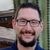# Extract Value Across Multiple Columns

819
7
09-16-2021 01:31 PMOccasional Contributor

Hello,

I have a value that can be housed in 4 different columns. Not only that but the value is within a text string. Here is an example of the string -

``1-12: ESCC02SPL01, 1-12``

I am trying to write an evaluation or batch calculation that searches the 4 columns for the value (contains 'SPL') and and populates a new column with the middle string (in between spaces) as the return. Such that the example above would evaluate to

``ESCC02SPL01``

Another detail is that I need the first instance of when SPL is in the value. So if it is present in columns 3 & 4, I need to take the value that is in column 3.

So far I am trying to put the columns in a list and then use Find to iterate over the list. I am more of a Python person so I'm not exactly sure how to do this with Arcade. What I have so far is this

``````var row1 = \$feature.f0;
var row2 = \$feature.f0spare;
var row4 = \$feature.f1

var rowlist = [row1, row2, row3, row4]

var splitter = Find('SPL', rowlist, 0)

if (splitter > 0) {
return splitter
};``````

But this is returning incorrect values.

Any help or opinions on this is greatly appreciated. Thanks for reading.

Tags (2)
1 Solution

Accepted SolutionsbyMVP Frequent Contributor
``````var values = [
\$feature.f0,
\$feature.f0spare,
\$feature.f1
]
for(var v in values) {
var value = values[v]
if(Count(value) > Count(Replace(value, "SPL", ""))) {
value = Replace(value, ":", "")
value = Replace(value, ",", "")
return Split(value, " ")
// you return the first found column, the next columns won't be evaluated.
}
}
// return a default value if no column contains a value with "SPL"
return null``````

Have a great day!
Johannes
7 RepliesNew Contributor II

What value is it returning for your example?Occasional Contributor

Find returns the index # of the string you supply it, so it wasn't quite right for what I was trying to do.by
New Contributor III

Instead of using a list to iterate over you could use the When() function in Arcade. This will let you test a series of conditional expressions. Then you can put your text logic as one of the returns for the expression(s).  I would also look into the Function Reference documentation for some other helpful functions to accomplish this>

```// Custom Arcade Expression for Symbology
// Test to see if "Y" is in any of the three exceedance columns. If so,the
// expression returns true and the point is symbololized as "Y". Otherwise, the expression
// returns false and the point is symbolized as "N".

// Eceedance Fields

var exc1 =  \$feature["As_1_Exc"]

var exc2 = \$feature["As_2_Exc"]

var exc3 = \$feature["As_3_Exc"]

// Testing which fields have a "Y" value and return True, else return False

var soilExceeds = When( exc1 == 'Y' || exc2 == 'Y' || exc3 == 'Y', 'Y', 'N')

//Return Values

return soilExceeds```Occasional Contributor

Okay I see. This is a great example, thank you. I'll just have to find the equivalent of str.contains for Arcade, then parse out the string I need based on a split index (which I think you can do in Arcade).by
New Contributor III

Great!

Yes, I think you could use the Split() function in Arcade to accomplish this?

Otherwise if you have a constant number of characters then maybe a Mid()function could be used to grab it?byMVP Frequent Contributor
``````var values = [
\$feature.f0,
\$feature.f0spare,
\$feature.f1
]
for(var v in values) {
var value = values[v]
if(Count(value) > Count(Replace(value, "SPL", ""))) {
value = Replace(value, ":", "")
value = Replace(value, ",", "")
return Split(value, " ")
// you return the first found column, the next columns won't be evaluated.
}
}
// return a default value if no column contains a value with "SPL"
return null``````

Have a great day!
JohannesOccasional Contributor

Wow this is great, thank you once again Johannes!